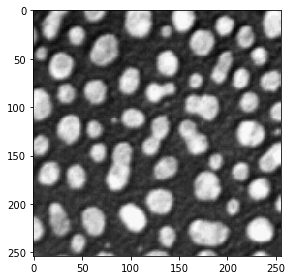# Functional parameters#

A core concept of the python language is functional programming: We define functions and apply them to our data.

import numpy as np

values = np.asarray([1, 2, 3, 4, 10])

def double_number(x):
return x * 2

double_number(values)

array([ 2,  4,  6,  8, 20])


In python you can also have variables that contain a function and can be executed:

my_function = double_number

my_function(values)

array([ 2,  4,  6,  8, 20])


## Custom functional parameters#

You can also define your custom functions taking functional parameters. For example, we can define a count_blobs function that takes an image and a threshold_algorithm-function as parameter.

import matplotlib.pyplot as plt
from skimage.measure import label

def count_blobs(image, threshold_algorithm):
# binarize the image using a given
# threshold-algorithm
threshold = threshold_algorithm(image)
binary = image > threshold

# show intermediate result
# plt.imshow(binary)

# return count blobs
labels = label(binary)
return labels.max()


We now open an image and analyse it twice.

from skimage.io import imread, imshow

imshow(blobs_image)

<matplotlib.image.AxesImage at 0x17968acd0>We now count the blobs in this image with two different algorithms we provide as parameter:

from skimage.filters import threshold_otsu

count_blobs(blobs_image, threshold_otsu)

64

from skimage.filters import threshold_yen

count_blobs(blobs_image, threshold_yen)

67


## Exercise#

Assume you want to find out which threshold algorithm works best for your image. Therefore, you may want to take a look at the image being thresholded by multiple algoritms. Define a list of threshold algorithms, e.g. from this list. Program a for-loop that applies the threshold algorithms to the blobs image and shows the results. The result should look similar to this example.